﻿ Circular motion and gravitation

# Circular motion

If an object moves on a circle, we call that object's motion circular motion. A ball on a string that swing in a circle, motion of the moon around the earth are examples of circular motion. We can also consider the motion of an object on a curved path as circular motion, if the path is an arc of a circle, because the object is in circular motion over that part of the circle.

Since the direction of motion of an object in circular motion changes continuously, the direction of velocity is different at different times (or points). At a given point on the path, the direction of velocity is tangent to the circle at that point.

#### Uniform circular motion

If the speed of an object in circular motion is constant, we call that uniform circular motion. Remember that, speed is the magnitude of velocity, although speed is constant, velocity is not constant, because the direction of velocity is changing. In this page, we focus our attention on uniform circular motion.

#### Centripetal force

According to Newton's first law, every object that is in motion continues its motion on a straight line when there is no external force acting on. Circular motion is not in a straight line. So, in order to make an object's motion circular (non-straight line), there must be a net external force applied on the object. That net external force is called centripetal force, $\vec F_c$. To keep an object in a circle, the applied force should point to the center of the circle at each and every points. So, the direction of the centripetal force is towards the center of the circle.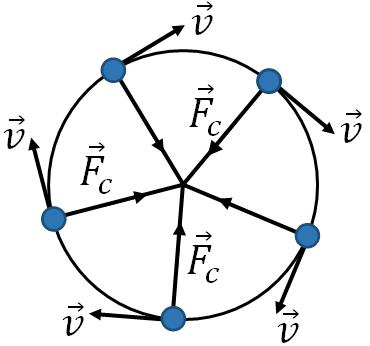Centripetal force acts toward the center always. Moon revolves around the earth in a nearly circular orbit, the force of gravity on the moon by the earth is the centripetal force of the moon.

#### Acceleration in uniform circular motion

Since there is a net force called centripetal force is acting on an object in circular motion, there must be an acceleration of the object according to Newton's second law. That acceleration, we call centripetal acceleration, $\vec a_c$. Since the direction of acceleration is the direction of the net force on the object, direction of centripetal acceleration is also towards the center of the circle.
Note that an object in uniform circular motion has an acceleration although the speed is constant, because velocity of the object is not constant.

Magnitude of the centripetal acceleration is (for derivation, refer the textbook),

$a_c=\dfrac{v^2}{r}$
where $v$ is the speed of the object and $r$ is the radius of the circle.

If $m$ is the mass of the object, then the centripetal force is,
$F_c=ma_c=\dfrac{mv^2}{r}$ [by Newton's second law]

#### Period of circular motion

If an object, continues the motion on a circle, it repeats the motion in certain time interval, that interval is the period of the motion. Period is the time taken by the object to complete one circle. In one period of time, the object travels a distance equal to the circumference of the circle, that is $2\pi r$.Therefore, the period, $T$ of circular motion is,

$T=\dfrac{2\pi r}{v}$

#### Unbanked and banked high way curves

There are two types of highway curves (roads), unbanked or horizontal curve and banked curve. Banked curve is advantageous during the snowy season as it provides better control against vehicles slide off.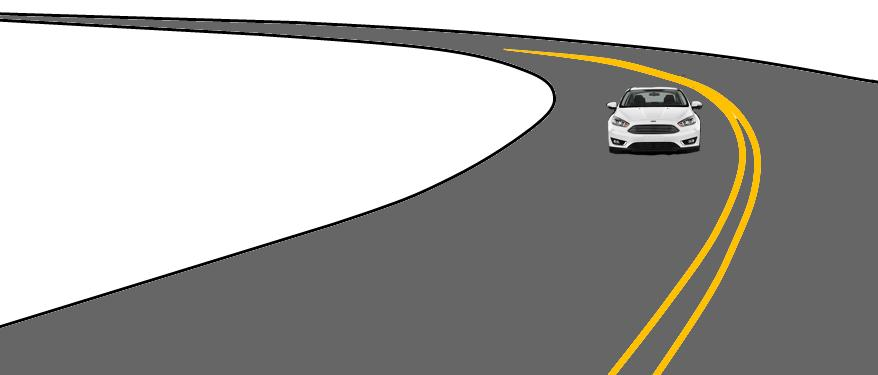A car on a horizontal high way curve.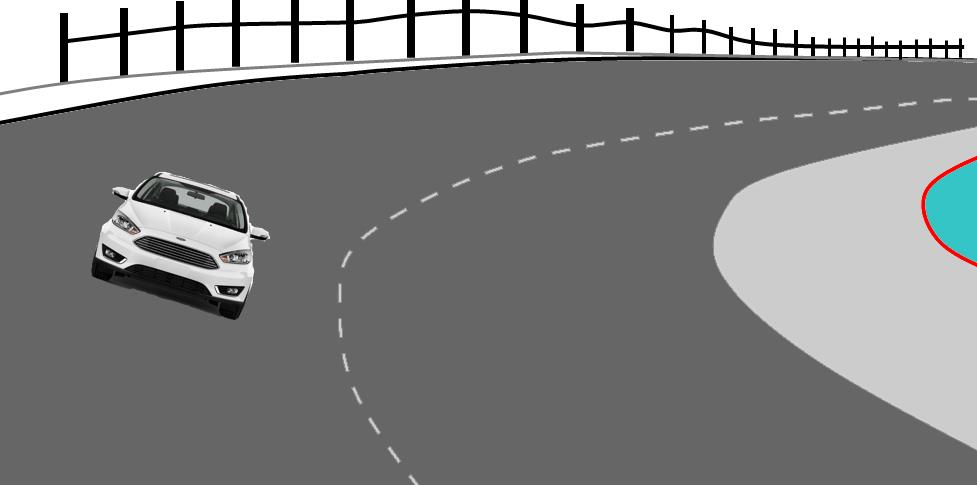A car on a banked highway curve.

#### Unbanked curve:

On an unbanked or horizontal curve, a car or truck follows circular path (or part of a circle). Since the vehicle is in circular motion, there must be an external force acting on it. On a horizontal road, the friction force on the tires by the road provide the required centripetal force. If there is no friction, a car cannot follow a horizontal curve as you know that it is very difficult to make a turn on a flat icy pavement. Therefore, on a banked curve,

$F_c=F_{fr}$

Now, the question is what type of friction provides the centripetal force on an unbanked flat road? is it static or kinetic ? Since friction is the centripetal force on a horizontal curve, the friction force's direction should be towards the center of the circle. But there is no motion of the vehicle in that direction, so the friction is static.

Depends upon the friction between the road and the tires, there is a maximum speed, above which a car cannot follow a curve or make a turn on a flat road. This maximum speed,$v_{max}$ can be obtained by equating centripetal force to the maximum available force of friction:

$\dfrac{mv_{max}^2}{r}=\mu_sF_n$.

If $m$ is the mass of the vehicle, then the normal force on the car, is $F_n=mg$. Substituting this in the above equation, and solving for $v_{max}$, we get,

$v_{max}=\sqrt{\mu_s rg}$

This is the maximum speed at which a car can safely turn on a flat road. If there is ice on the road, then $\mu_s$, decreases that reduces the safe speed. The maximum speed is independent of the mass of the vehicle.

#### Banked curve

A banked curve is tilted above the horizontal by an angle, usually towards the inside of the curve. The angle of the tilt is called banking angle. If you make a circle out of the curved path of a car, it is a horizontal circle. So, for the car to follow the curve, there must be a horizontal force acting on it towards the center of the circle, that is the centripetal force. How can we have a horizontal force on the car so that it can follow the curve?. If you look at the normal force on the car, it is not vertical. That means, there is a horizontal component of the normal force. This component of the normal force acts as the required centripetal force for the car to follow the curve.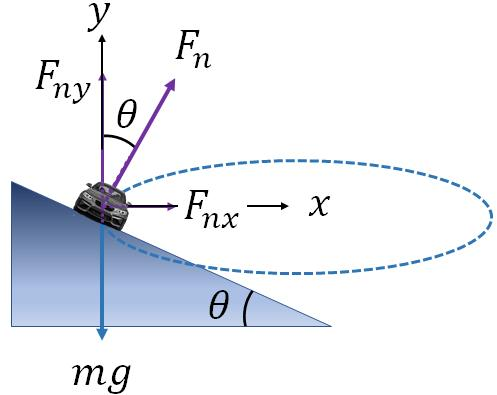A car on a banked curve has the $x$ component of normal force towards the center.

Let us take the $x$ axis towards the center of the circle and the $y$ axis vertical. From the geometry of the figure, you can see that the normal force is at an angle $\theta$ from the vertical. Finding the $x$ and the $y$ components of the normal force, we have

$F_{nx}=F_n \sin\theta$ and

$F_{ny}=F_n \cos\theta$

Since the $x$ component of the normal force is the centripetal force, we can write

$F_n \sin\theta=\dfrac{mv^2}{r}$

From the figure, normal force does not balance the force of gravity, $mg$, but the $y$ component of the normal force does. Therefore,

$F_n \cos\theta=mg$.

Dividing the previous equation by this equation, we get,

$\tan \theta = \dfrac{v^2}{rg}$

Solving for $v$,

$v=\sqrt{rg\tan\theta}$

This $v$ is called the design speed or the safe speed to drive on a banked curve. Design speed is independent of the mass of the vehicle.
Note that, when deriving the above equation we ignore the friction force on the tires by the road.

#### Law of universal gravitation:

The law of universal gravitation was published by Isaac Newton in 1687. It states that

"every particle in the universe attracts every other particle with a force, which is proportional to the product of their masses and inversely proportional to the square of the distance between them."

If there are two objects, they attract each other with a force called gravitational force. The magnitude of the gravitational force between two objects with masses $m_1$ and $m_2$ is

$F_g=G\dfrac{m_1m_2}{r^2}$

where $r$ is the distance between the two objects, it is measured from the center to center of the objects; and $G=6.67\times10^{-11}Nm^2/kg^2$ is a constant, called, the gravitational constant.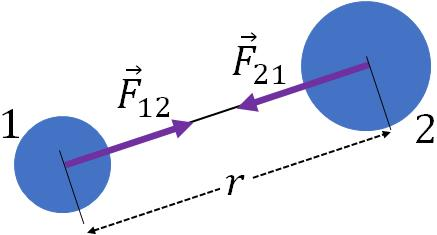Gravitational force between two objects. Gravitational force is always attractive. It decreases as the distance between the object increases as $1/r^2$.

In the figure, you see that, object 1 is exerting a gravitational force on object 1 and the object 2 is exerting an equal amount of gravitational force on object 2, but in the opposite direction, according to Newton's third law.

#### Acceleration due to gravity on a planet:

You already learned that near the surface of the earth, acceleration due to gravity, $g$ is $9.8 m/s^2$. Acceleration due to gravity decreases with altitude.

Now, we are going to derive an equation for acceleration due to gravity on a planet or on a celestial object. Let $M_p$ is the mass of the planet or the celestial object. Assume that you want to find the acceleration due to gravity at a point P, that is at a distance $r$ from the planet (this the distance from the center of the planet). To do that, we imagine that there is a test object of mass, $m$ at P.

Let $g$ is the acceleration due to gravity of the object at the point P. So, the force of gravity on the test object is

$F_g=mg$

You can also find the force of gravity, i.e., the gravitational force on the object by the planet using the law of universal gravitation,

$F_g=G\dfrac{mM_p}{r^2}$.

Now, equate the two equations above and solve for $g$, you will get,

$g=\dfrac{GM_p}{r^2}$.

Acceleration due to gravity depends on the planet's mass and the distance from its center. It decreases with distance as $1/r^2$, an inverse square law. On the surface of the moon, $g$ is about one-sixth that of the earth, because of its smaller mass.

### Satellites

Satellites revolve around the earth in nearly circular orbits. Man made satellites are used for various applications from weather forecasting to navigation. Since a satellite is in circular motion, there must be a centripetal force acting on the satellite. That centripetal force is actually the gravitational force on the satellite by the earth.

If $m$ is the mass of a satellite that is revolving around the earth, then the gravitational force on the satellite by the earth is

$F_g=G\dfrac{M_E\,m}{r^2}$.

Since this is the centripetal force, we have

$\dfrac{mv^2}{r}=G\dfrac{M_E\,m}{r^2}$.

where $r$ is the radius of the orbit, i.e., the distance of the satellite from the center of the earth.

Solving for $v$,we get,

$v=\sqrt{\dfrac{GM_E}{r}}$.

In the above equation, there is no $m$, the mass of the satellite, so the speed of a satellite is independent of its mass. Speed depends only on the distance $r$. It varies as $1/r^2$, an inverse square law, closer the satellite to the earth, faster it moves.

#### Geosynchronous satellites:

Satellites that appear to stay at the same spot above the earth are called a geosynchronous satellites. For a satellite to be geosynchronous, it should meet two conditions: (i) the orbital period of the satellite should be same as the earth's rotational period, and (ii) the orbit should be exactly aligned with the equator of the earth. So, the period of a geosynchronous satellite is 1 day, the earth's rotational period. Since a geosynchronous satellite appears to be fixed at one spot above the earth, they are used for satellite television broadcasts and communication purposes. Based on the period, a geosynchronous satellite orbits the earth at a height of approximately 36,000 km with a speed of about 2800 km/h.

#### Weightlessness:

We all feel weight due to a normal force acting on us. When there is no normal force, we feel weightlessness. You can realize this by standing in an elevator. When the elevator accelerates downward, you feel less weight, and when it accelerates upward you feel more. The weight that you feel is the normal force (also called apparent weight) exerted on you by the surface of the elevator. As you already learned in "forces", the normal force on a person in an elevator is

$F_{n}-mg=ma$

where $a$ is the acceleration of the elevator (or the person).

If the elevator cable suddenly breaks, then the elevator and the person are in free fall. Since the person is in free fall, her/his acceleration, is $a=-g$. If you substitute this in the above equation, you get $F_{n}=0$, and the person feels weightlessness. An astronomer in a spacecraft is also in free fall along with the spacecraft as gravity is the only force acting on the astronomer or the spacecraft, so he/she feels weightlessness. Note that one feel weightless due to the absence of normal force. Although apparent weight is zero during free fall, it doesn't mean that the actual weight of the person is zero, that is still $mg$.

#### Planetary motion:

All the planets including earth revolve around the sun. There are three laws developed by Kepler’s that describe the motion of planets around the sun. These laws were developed in the 1600’s, even before the invention of telescope and also about 50 years before the Newton’s laws.

#### Kepler’s first law:

Each planet revolves around the Sun in an elliptical path with the Sun at one focus.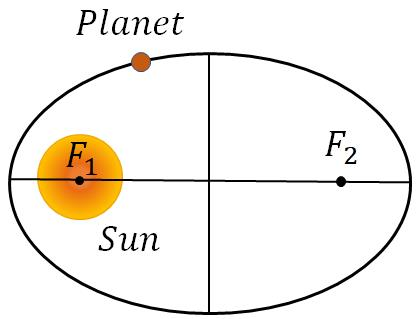A planet revolves around the Sun in an elliptical orbit. $F_1$ and $F_2$ are the focal points of the ellipse.

#### Kepler’s second law:

An imaginary line drawn from the Sun to a planet sweeps out equal areas in equal times.
In the figure, the shaded areas 1 and 2, are the same if the time taken by the planet to move from A to B is same as that from C to D.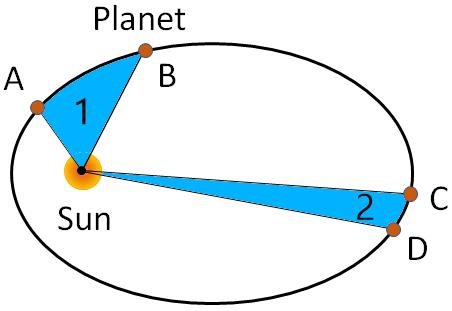A planet sweeps out equal areas in equal times.

#### Kepler’s third law:

The ratio of the squares of the periods of any two planets revolving around the sun is equal to the ratio of the cubes of their mean distances from the sun.
$\bigg(\dfrac{T_1}{T_2}\bigg)^2=\bigg(\dfrac{s_1}{s_2}\bigg)^3$ where $T_1$ and $T_2$ are the periods of planets 1 and 2 and $s_1$ and $s_2$ are their distances from the sun. For circular orbits, $s=r$, the radius of the orbit.